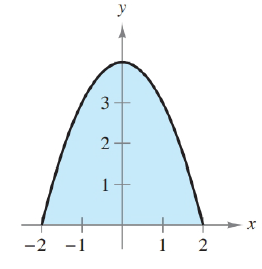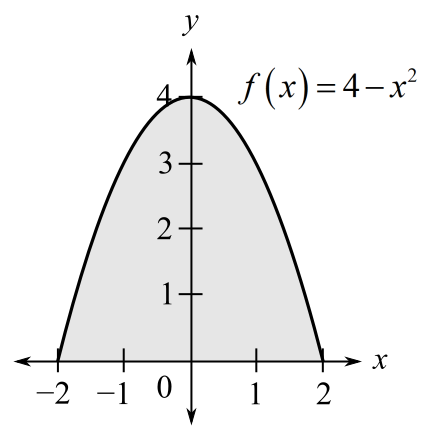Chapter 5, Problem 53RE### Calculus: An Applied Approach (Min...

10th Edition
Ron Larson
ISBN: 9781305860919

#### Solutions

Chapter
Section### Calculus: An Applied Approach (Min...

10th Edition
Ron Larson
ISBN: 9781305860919
Textbook Problem
1 views

# Finding Area by the Fundamental Theorem In Exercises 53-58, find the area of the region. f ( x ) = 4 − x 2To determine

To calculate: The area of region bounded by graph of function f(x)=4x2Explanation

Given Information:

The provided graph of function f(x)=4x2.

Formula used:

Area of function f(x) bound from x=a and x=b is given by

Area=abf(x)dx

The fundamental theorem of calculus:

abf(x)dx=F(b)F(a)

Here, F is function such that F(x)=f(x) for all x in [a,b].

Calculation:

The provided graph of function f(x)=4x2.

The graph of function f(x)=4x2 is parabola bounded from x=2 and x=2. The integration of function f(x)=4x2 from x=2 and x=2 represent the area of region bound graph

### Still sussing out bartleby?

Check out a sample textbook solution.

See a sample solution

#### The Solution to Your Study Problems

Bartleby provides explanations to thousands of textbook problems written by our experts, many with advanced degrees!

Get Started

#### In Exercises 63-68, use the graph of the function f to determine limxf(x) and limxf(x) 67.

Applied Calculus for the Managerial, Life, and Social Sciences: A Brief Approach

#### In problems 1-16, solve each equation. 7. Solve

Mathematical Applications for the Management, Life, and Social Sciences

#### Multiply. (45y)(4+5y)

Trigonometry (MindTap Course List)

#### True or False: is monotonic.

Study Guide for Stewart's Single Variable Calculus: Early Transcendentals, 8th

#### Explain the distinction between science and pseudoscience.

Research Methods for the Behavioral Sciences (MindTap Course List)